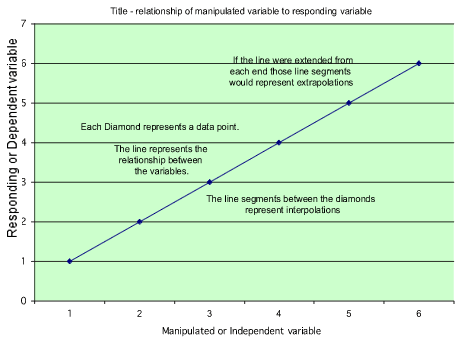Data analysis, variables, relationships, and graphing

A good experiment attempts to control all variables except the variable(s) that are being manipulated to see if a change can be observed and a cause and effect relationship can be reasoned.

Variable is a property or condition that can change.
A variable may or may not cause a significant change.

Manipulated variable is the variable that the experimenter decides to change to see if there is or is not an effect.

Responding variable is the variable that will change as a result of the change in the manipulated variable. It may also be observed and or measured to determine the quantity or quality of change.

When we plot information on a graph the manipulated variable always is plotted on the X - axis and the responding variable is always plotted on the Y - axis.

Independent variable is another name for manipulated variable. It is independently selected by the experimenter to be manipulated.

Dependent variable is watched by the experimenter and will respond to the manipulated or independent variable if there is a relationship.

These relationships can be represented on a chart or graph: Before deciding to use a chart or graph a person needs to decide if the data is continuous or categorical

Continuous is data that can be represented on a continuum with infinite possibilities. Temperature for example can be represented by whole numbers, decimal numbers, and fractions.

Categorical is data that is represented by a limited number of examples (discrete, finite, not continuous). Temperature could be categorical if it were categorized into hot, medium, or cold. Kinds of vehicles, people's favorite food... One might argue that favorite foods could be continuous or infinite. However, it can be represented by categories and a category labeled others. Therefore, limiting the possibilities.

Continuous data is best shown on a line graph and categorical in bar, pie, or other kinds of charts.

Extrapolation is a prediction that is made from outside (extra) the data points collected and represented on the graph.

Interpolation is a prediction that is made between the data points (inter, like interstate, between or among states).

Relationship is the way two or more variables, concepts, objects, or people are connected.